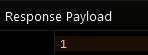# Get Object Count¶

The Object Count API provides a way to obtain the following values from the server:

• Number of objects in a table
• Number of objects matching query
• Number of related objects

Retrieving object count for a table/query can be done with a 'data store' object. A reference to a data store can be obtained using the following code:

``````// obtaining DataStore for the NSDictionary approach
MapDrivenDataStore *dataStore = [Backendless.shared.data ofTable:@"TABLE-NAME"];

// obtaining DataStore for the custom-class approach
DataStoreFactory *dataStore = [Backendless.shared.data of:[YOUR-CLASS class]];
``````
``````// obtaining DataStore for the NSDictionary approach
let dataStore = Backendless.shared.data.ofTable("TABLE-NAME")

// obtaining DataStore for the custom-class approach
let dataStore = Backendless.shared.data.of(YOUR-CLASS.self)
``````

where

Argument                Description
`YOUR-CLASS` identifies a table on the server.
`"TABLE-NAME"` the name of the table for which to get the data store. All subsequent data store operations will be performed in the specified table.

Important

All Data Service methods in Backendless must be accessed through data stores.

A data store object provides the following APIs for retrieving object counts in Backendless:

``````// get total number of objects in a table identified by the dataStore object
- (void)getObjectCountWithResponseHandler:^(NSNumber * _Nonnull)responseHandler errorHandler:^(Fault * _Nonnull)errorHandler;

// get number of objects for a query in the table identified by the dataStore object
- (void) getObjectCountWithQueryBuilder:(DataQueryBuilder * _Nonnull)queryBuilder responseHandler:^(NSNumber * _Nonnull)responseHandler errorHandler:^(Fault * _Nonnull)errorHandler;
``````
``````// get total number of objects in a table identified by the dataStore object
func getObjectCount(responseHandler: ((NSNumber) -> Void)!, errorHandler: ((Fault) -> Void)!)

// get number of objects for a query in the table identified by the dataStore object
func getObjectCount(queryBuilder: DataQueryBuilder, responseHandler: ((NSNumber) -> Void)!, errorHandler: ((Fault) -> Void)!)
``````
``````// get total number of objects in a table identified by the dataStore object
- (void)getObjectCountWithResponseHandler:^(NSNumber * _Nonnull)responseHandler errorHandler:^(Fault * _Nonnull)errorHandler;

// get number of objects for a query in the table identified by the dataStore object
- (void) getObjectCountWithQueryBuilder:(DataQueryBuilder * _Nonnull)queryBuilder responseHandler:^(NSNumber * _Nonnull)responseHandler errorHandler:^(Fault * _Nonnull)errorHandler;
``````
``````// get total number of objects in a table identified by the dataStore object
func getObjectCount(responseHandler: ((NSNumber) -> Void)!, errorHandler: ((Fault) -> Void)!)

// get number of objects for a query in the table identified by the dataStore object
func getObjectCount(queryBuilder: DataQueryBuilder, responseHandler: ((NSNumber) -> Void)!, errorHandler: ((Fault) -> Void)!)
``````

where:

Argument                Description
`responseHandler` a block (closure) to handle successful result of an asynchronous call.
`errorHandler` a block (closure) to handle fault result of an asynchronous call.

#### Return Value¶

The API returns the object count as a result of the request.

#### Examples¶

The following sample request retrieves total number of objects in table Order:

``````DataStoreFactory *dataStore = [Backendless.shared.data of:[Order class]];
[dataStore getObjectCountWithResponseHandler:^(NSNumber *objectCount) {
NSLog(@"Total objects in the Order table = %@", objectCount);
} errorHandler:^(Fault *fault) {
NSLog(@"Error: %@", fault.message);
}];
``````
The following sample request retrieves total number of objects in table Order which satisfy the condition of orderAmount > 100:
``````DataQueryBuilder *queryBuilder = [DataQueryBuilder new];
[queryBuilder setWhereClauseWithWhereClause:@"orderAmount > 100"];

DataStoreFactory *dataStore = [Backendless.shared.data of:[Order class]];
[dataStore getObjectCountWithQueryBuilder:queryBuilder responseHandler:^(NSNumber *objectCount) {
NSLog(@"Objects with orderAmount > 100 in the Order table = %@", objectCount);
} errorHandler:^(Fault *fault) {
NSLog(@"Error: %@", fault.message);
}];
``````
The following sample request retrieves total number of related "child" objects for a parent object. The parent table is Person. It contains a relation column called address pointing to the Address table. The query below retrieves a count of related child objects for a parent object with objectID of XXXXX. The whereClause query syntax for this scenario is:
``````Person[address].objectId = 'XXXXX'
``````

``````DataQueryBuilder *queryBuilder = [DataQueryBuilder new];

DataStoreFactory *dataStore = [Backendless.shared.data of:[Address class]];
[dataStore getObjectCountWithQueryBuilder:queryBuilder responseHandler:^(NSNumber *objectCount) {
NSLog(@"Related objects count = %@", objectCount);
} errorHandler:^(Fault *fault) {
NSLog(@"Error: %@", fault.message);
}];
``````

The following sample request retrieves total number of objects in table Order:

``````let dataStore = Backendless.shared.data.of(Order.self)
dataStore.getObjectCount(responseHandler: { objectCount in
print("Total objects in the Order table = \(objectCount)")
}, errorHandler: { fault in
print("Error: \(fault.message ?? "")")
})
``````
The following sample request retrieves total number of objects in table Order which satisfy the condition of orderAmount > 100:
``````let queryBuilder = DataQueryBuilder()
queryBuilder.setWhereClause(whereClause: "orderAmount > 100")

let dataStore = Backendless.shared.data.of(Order.self)
dataStore.getObjectCount(queryBuilder: queryBuilder, responseHandler: { objectCount in
print("Objects with orderAmount > 100 in the Order table = \(objectCount)")
}, errorHandler: { fault in
print("Error: \(fault.message ?? "")")
})
``````
The following sample request retrieves total number of related "child" objects for a parent object. The parent table is Person. It contains a relation column called address pointing to the Address table. The query below retrieves a count of related child objects for a parent object with objectID of XXXXX. The whereClause query syntax for this scenario is:
``````Person[address].objectId = 'XXXXX'
``````

``````let queryBuilder = DataQueryBuilder()

dataStore.getObjectCount(queryBuilder: queryBuilder, responseHandler: { objectCount in
print("Related objects count = \(objectCount)")
}, errorHandler: { fault in
print("Error: \(fault.message ?? "")")
})
``````

The following sample request retrieves total number of objects in table Order:

``````MapDrivenDataStore *dataStore = [Backendless.shared.data ofTable:@"Order"];
[dataStore getObjectCountWithResponseHandler:^(NSNumber *objectCount) {
NSLog(@"Total objects in the Order table = %@", objectCount);
} errorHandler:^(Fault *fault) {
NSLog(@"Error: %@", fault.message);
}];
``````
The following sample request retrieves total number of objects in table Order which satisfy the condition of orderAmount > 100:
``````DataQueryBuilder *queryBuilder = [DataQueryBuilder new];
[queryBuilder setWhereClauseWithWhereClause:@"orderAmount > 100"];

MapDrivenDataStore *dataStore = [Backendless.shared.data ofTable:@"Order"];
[dataStore getObjectCountWithQueryBuilder:queryBuilder responseHandler:^(NSNumber *objectCount) {
NSLog(@"Objects with orderAmount > 100 in the Order table = %@", objectCount);
} errorHandler:^(Fault *fault) {
NSLog(@"Error: %@", fault.message);
}];
``````
The following sample request retrieves total number of related "child" objects for a parent object. The parent table is Person. It contains a relation column called address pointing to the Address table. The query below retrieves a count of related child objects for a parent object with objectID of XXXXX. The whereClause query syntax for this scenario is:
``````Person[address].objectId = 'XXXXX'
``````

``````DataQueryBuilder *queryBuilder = [DataQueryBuilder new];

[dataStore getObjectCountWithQueryBuilder:queryBuilder responseHandler:^(NSNumber *objectCount) {
NSLog(@"Related objects count = %@", objectCount);
} errorHandler:^(Fault *fault) {
NSLog(@"Error: %@", fault.message);
}];
``````

The following sample request retrieves total number of objects in table Order:

``````let dataStore = Backendless.shared.data.ofTable("Order")
dataStore.getObjectCount(responseHandler: { objectCount in
print("Total objects in the Order table = \(objectCount)")
}, errorHandler: { fault in
print("Error: \(fault.message ?? "")")
})
``````
The following sample request retrieves total number of objects in table Order which satisfy the condition of orderAmount > 100:
``````let queryBuilder = DataQueryBuilder()
queryBuilder.setWhereClause(whereClause: "orderAmount > 100")

let dataStore = Backendless.shared.data.ofTable("Order")
dataStore.getObjectCount(queryBuilder: queryBuilder, responseHandler: { objectCount in
print("Objects with orderAmount > 100 in the Order table = \(objectCount)")
}, errorHandler: { fault in
print("Error: \(fault.message ?? "")")
})
``````
The following sample request retrieves total number of related "child" objects for a parent object. The parent table is Person. It contains a relation column called address pointing to the Address table. The query below retrieves a count of related child objects for a parent object with objectID of XXXXX. The whereClause query syntax for this scenario is:
``````Person[address].objectId = 'XXXXX'
``````

``````let queryBuilder = DataQueryBuilder()

dataStore.getObjectCount(queryBuilder: queryBuilder, responseHandler: { objectCount in
print("Related objects count = \(objectCount)")
}, errorHandler: { fault in
print("Error: \(fault.message ?? "")")
})
``````

### Codeless Reference¶where:

Argument                Description
`table name` Name of the data table where to calculate the object count.
`where clause` Optional argument. If set, it is a search query used by the server it to determine the number of objects matching the condition. Refer to the Search With The Where Clause topic for more information.
`distinct` Used to return unique objects from the data table. Only custom properties/columns are considered in the query. Hence, if the data table contains a duplicate object, then it is not counted in the operation.

Returns the number of objects in a table (if the `where clause` is not set), otherwise the number of objects matching the search query.

Consider the following records in the `employees` data table: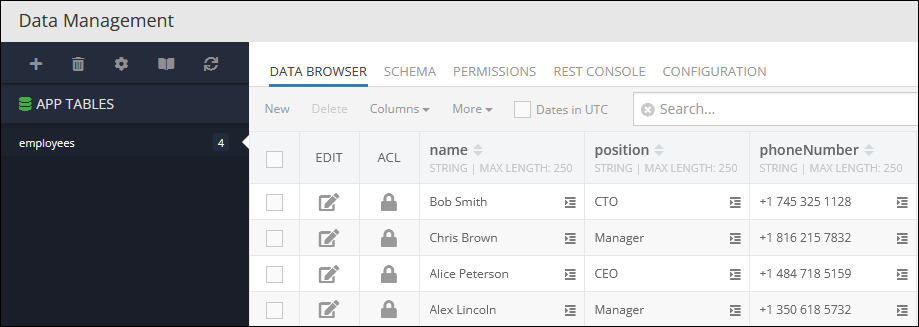The example below counts specific objects in the data table since the `where clause` condition is set to exclude objects that contain the value `'Manager'` in the column `position`. Thereby, objects with the `'Manager'` values in the `position` column are not counted in the operation.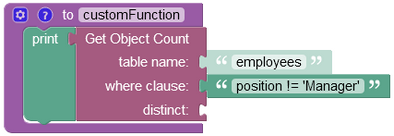The result of this operation will look as shown below after the Codeless logic runs: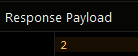Consider the data table below that contains two duplicate objects: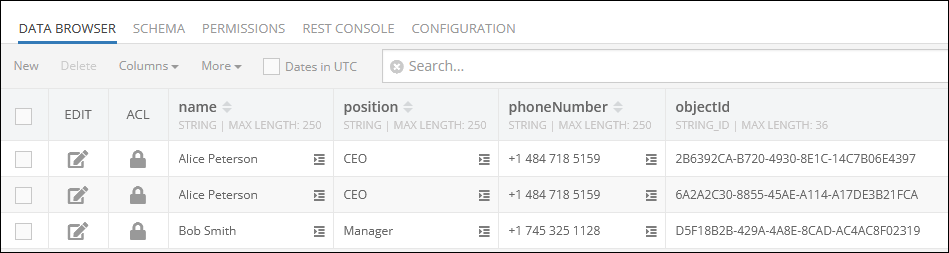The Codeless logic below has a `where clause` condition which is set to include in the operation only those objects that contain the value `'CEO'` in the `position` column. The `distinct` parameter is set to `true`, hence the operation is set to count only unique objects, duplicates are skipped.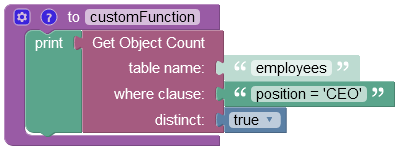The result of this operation will look as shown below after the Codeless logic runs: